Download Presentation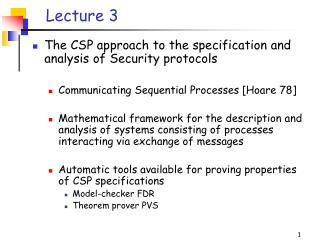Lecture 3

# Lecture 3 - PowerPoint PPT Presentation

Lecture 3. The CSP approach to the specification and analysis of Security protocols Communicating Sequential Processes [Hoare 78] Mathematical framework for the description and analysis of systems consisting of processes interacting via exchange of messagesI am the owner, or an agent authorized to act on behalf of the owner, of the copyrighted work described.
Download Presentation## Lecture 3

An Image/Link below is provided (as is) to download presentation

Download Policy: Content on the Website is provided to you AS IS for your information and personal use and may not be sold / licensed / shared on other websites without getting consent from its author.While downloading, if for some reason you are not able to download a presentation, the publisher may have deleted the file from their server.

- - - - - - - - - - - - - - - - - - - - - - - - - - E N D - - - - - - - - - - - - - - - - - - - - - - - - - -
Presentation Transcript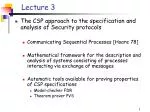Lecture 3
• The CSP approach to the specification and analysis of Security protocols
• Communicating Sequential Processes [Hoare 78]
• Mathematical framework for the description and analysis of systems consisting of processes interacting via exchange of messages
• Automatic tools available for proving properties of CSP specifications
• Model-checker FDR
• Theorem prover PVS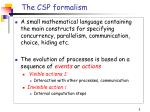The CSP formalism
• A small mathematical language containing the main constructs for specifying concurrency, parallelism, communication, choice, hiding etc.
• The evolution of processes is based on a sequence of events oractions
• Visible actions S
• Interaction with other processes, communication
• Invisible action t
• Internal computation steps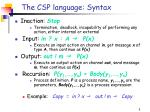The CSP language: Syntax
• Inaction: Stop
• Termination,deadlock, incapability of performing any action, either internal or external
• Input: in ? x : A g P(x)
• Execute an input action on channel in, get message x of type A, then continue as P(x)
• Output: out ! m g P(x)
• Execute an output action on channel out, send message m, then continue as P(x)
• Recursion: P(y1,…,yn) = Body(y1,…,yn)
• Process definition. P is a process name, y1,…,yn are the parameters, Body(y1,…,yn) is a process expression
• Example: Copy = in ? x g out ! m g Copy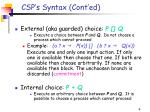CSP’s Syntax (Cont’ed)
• External (aka guarded) choice: P [] Q
• Execute a choice between P and Q. Do not choose a process which cannot proceed
• Example: (a ? x g P(x)) [](b ? x g Q(x))

Execute one and only one input action. If only one is available then choose that one. If both are available than choose arbitrarily. If none are available then block. The unchoosen branch is discarded (commitment)

• Internal choice: P + Q
• Execute an arbitrary choice between P and Q. It is possible to choose a process which cannot proceed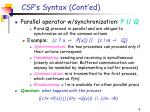CSP’s Syntax (Cont’ed)
• Parallel operator w/synchronization: P || Q
• P and Q proceedin parallel and are obliged to synchronize on all the common actions
• Example: (c ? x g P(x)) ||(c ! m g Q)
• Synchronization: the two processes can proceed only if their actions correspond
• Handshaking: sending and receiving is symultaneous (clearly an abstraction. Buffered communication can anyway be modeled by implementing a buffer process)
• Communication: m is transmitted to the first process, which continues as P(m).
• Broadcasting: c ! m is available for other parallelprocs
• Question: what happens with the process

((c?xgP(x)) [] (d?y gQ(y))) || (c!m gR)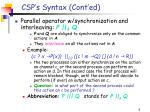CSP’s Syntax (Cont’ed)
• Parallel operator w/synchronization and interleaving: P ||A Q
• P and Q are obliged to synchronize only on the common actionsin A
• They interleave on all the actions not in A
• Example:

(c ? x gP(x)) ||{c} ((c ! m gQ) [] (d ! n g R))

• the two processes can either synchronize on the action on channel c, or the second process can perform an action on d. In this second case the first process will remain blocked, though, until the second will decide to perform (if ever) an output action on c.
• Question: in what part of the second process could this action on c be performed ?
• Abbreviation:P ||| Q stands for P ||f Q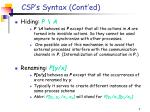CSP’s Syntax (Cont’ed)
• Hiding: P \ A
• P \A behaves as P except that all the actions in A are turned into invisible actions. So they cannot be used anymore to synchronize with other processes.
• One possible use of this mechanism is to avoid that external processes interfere with the communication channels in P. (Internalization of communication in P.)
• Renaming: P[y/x]
• P[x/y] behaves as P except that all the occurrences of x are renamed by y.
• Typically it serves to create different instances of the same process scheme
• Abbr: P[y1,y2 /x1,x2] will stand for P[y1/x1][y2/x2]Modeling Security Protocols in CSP
• Security protocols work through the interaction of a number of processes in parallel that send messages to each other. CSP is therefore an obvious notation for describing the participants and their role in the protocol
• Example: The Yahalom protocol
• Message 1 a g b : a.na
• Message 2 b g s : b.{a.na.nb}ServerKey(b)
• Message 3 s g a : {b. kab.na.nb}ServerKey(a) .{a.kab}ServerKey(b)
• Message 4 a g b : {a. kab}ServerKey(b) .{nb}kab
• Questions:
• Is the it based on symmetric or asymmetric cryptography?
• what arenaand nb, andwhat is their purpose?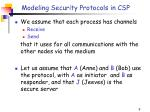Modeling Security Protocols in CSP
• We assume that each process has channels
• Send

that it uses for all communications with the other nodes via the medium

• Let us assume that A (Anne) and B (Bob) use the protocol, with A as initiator and B as responder, and that J (Jeeves) is the secure server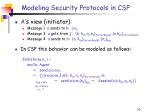Modeling Security Protocols in CSP
• A’s view (initiator):
• Message 1 a sends to b: a.na
• Message 3 a gets from ‘j’: {b. kab.na.nb}ServerKey(a) .{a.kab}ServerKey(b)
• Message 4 a sends to b: {a. kab}ServerKey(b) .{nb}kab
• In CSP this behavior can be modeled as follows:

Initiator(a,na ) =

env?b: Agent

g send.a.b.a.na

g [](receive.J.a{b. kab.na.nb}ServerKey(a) .m

kabe Key g send.a.b.m.{nb}kabg Session(a,b,kab,na,nb) )

nbe Nonce

m e TModeling Security Protocols in CSP
• B’s view (responder):
• Message 1 b getss from ‘a’: a.na
• Message 2 b sends to j: b.{a.na.nb}ServerKey(b)
• Message 4 b gets from ‘a’: {a. kab}ServerKey(b) .{nb}kab
• In CSP this behavior can be modeled as follows:

Responder(b,nb ) =

[](receive.a.b.a.na g send.b.J.b .{a.na.nb}ServerKey(b)

kabe Key g receive.a.b.{a. kab}ServerKey(b) .{nb}kab

nbe Nonce g Session(b,a,kab,na,nb) )

m e TModeling Security Protocols in CSP
• J’s view (server):
• Message 2 j gets from ‘b’: b.{a.na.nb}ServerKey(b)
• Message 3 j sends to a : {b. kab.na.nb}ServerKey(a) .{a.kab}ServerKey(b)
• In CSP this behavior can be modeled as follows:

Server(J,kab ) =

A,B e Agent g send.J.a. {b. kab.na.nb}ServerKey(a) .{a.kab}ServerKey(b)

Nb ,nbe Nonce g Server(J,ks ) )

Server(J) = ||| Server(J,kab )

kabe KeysServer

Question: why several server processes in parallel?Modeling an intruder
• We want to model an intruder that represents all potential intruder behaviors

Intruder(X) = learn?m: messages gIntruder(close(X U {m})

[]

say!m: X /\ messages gIntruder(X)

• Close(X) represents all the possible information that the attacker can infer from X.Typically we assume
• {k,m} |- encript(k,m)
• {encript(k,m), k-1} |- m
• {Sq<x1,…,xn>} |- xi
• {x1,…,xn} |- Sq<x1,…,xn>}Putting the network together

|||

|||

|||

Intruder(f)[take.x.y,fake.x.y/learn,say]

Bob

send

Jeeves

Anne

fake.x.Bob

send

send

say

Yves

learn

take.Anne.yAlternative with direct channels

Initiator(Anne,nA)[S]

|||

Responder(Bob,nB)[S]

|||

Server(Jeeves)[S]

|||

Intruder(f)[S]

Bob

send

Comm.Anne.Bob

Jeeves

Anne

fake.x.Bob